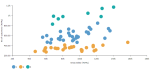# MLLIB.GMMCLUSTER(imputer, n_clusters, columns)

A Gaussian Mixture Model represents a composite distribution where points are drawn from one of K Gaussian sub-distributions, each with its own probability. It uses the expectation-maximization algorithm to induce the maximum-likelihood model given a set of samples.

###### Parameters
• imputer – strategy for dealing with null values:

• 0 – Replace null values with ‘0'

• 1 – Assign null values to a designated ‘-1' cluster

• n_clusters – Number of clusters which the algorithm should find, integer.

• columns – Dataset columns or custom calculations.

Example: MLLIB.GMMCLUSTER(0, 3, sum([Gross Sales]), sum([No of customers])) used as a calculation for the Color field of the Scatterplot visualization.###### Input data
• Size of input data is not limited.
• Without missing values.
• Character variables are transformed to numeric with label encoding.
###### Result
• Column of integer values starting with 0, where each number corresponds to a cluster assigned to each record (row) by the GMM algorithm.
###### Key usage points
• Cluster assignment is very flexible, clusters do not have to be spherical or have similar density.
• It allows for mixed membership of data points to clusters (data point belongs to each cluster, but to a different degree), where depending on the task could be more appropriate.
###### Drawbacks
• The algorithm may diverge and find solutions with infinite likelihood unless covariances are regularized.
###### Example

For the whole list of algorithms, see Data science built-in algorithms.